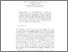# On Eccentric Connectivity Index of Eccentric Graph of Regular Dendrimer

Nagar, Atulya and Sastha, Sriram (2016) On Eccentric Connectivity Index of Eccentric Graph of Regular Dendrimer. Mathematics in Computer Science. ISSN 1661-8270Preview
Text
dendrimer.pdf

The eccentric connectivity index $$\xi ^c(G)$$ of a connected graph G is defined as $$\xi ^c(G) =\sum _{v \in V(G)}{deg(v) e(v)},$$ where deg( v) is the degree of vertex v and e( v) is the eccentricity of v. The eccentric graph, $$G_e$$, of a graph G has the same set of vertices as G; with two vertices u; v adjacent in Ge if and only if either u is an eccentric vertex of v or v is an eccentric vertex of u: In this paper, we obtain a formula for the eccentric connectivity index of the eccentric graph of a regular dendrimer. We also derive a formula for the eccentric connectivity index for the second iteration of eccentric graph of regular dendrimer.View Item